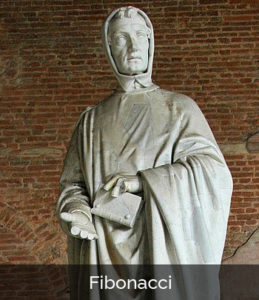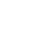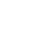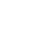# Fibonacci (Leonardo Pisano Bigollo)Fibonacci is best known for introducing Hindu-Arabic numerals to Europe which eventually superseded Roman numerals in everyday life. He is also known for the so-called “Fibonacci sequence” and his work in understanding the nature of number structures. When Fibonacci rose to prominence, Europe was recovering from the relatively long five-hundred-year period of the Dark Ages. Its cities were beginning to flourish again and trade started to increase rapidly. In short, the context was highly favourable for the arrival of a mathematician with Fibonacci’s talent.

### Early life and family

Fibonacci was, in fact, a nickname; the mathematician’s true name was Leonardo Pisano and he sometimes used the name Bigollo. He was born around 1170 AD into the Bonacci family, probably in the town of Pisa in Italy where he grew up.

His father, Guilielmo, was a merchant who became a senior customs official. Guilielmo was given a post in the town of Bugia (this would now be part of Algeria), North Africa and Fibonacci soon followed him across the sea. Fibonacci received his education in this area of Africa which was an integral part of the Arab world.

This means that he would have learned in Arabic and this must have drawn him into their intellectual world. Subsequently, he was given the opportunity by his father to travel extensively and explore this interest in greater depth. Fibonacci travelled to Syria, Egypt and then back to Greece.

During his travels, which were more akin to a research trip, Fibonacci discovered various mathematical texts that had been translated into Arabic, including the Hindu number system and its decimal approach.

Fibonacci immediately recognised the superiority of this system compared to the Roman numerals with which he had been familiar. He gathered a wealth of mathematical information and brought it back to Italy.

### Numbers

In Fibonacci’s 1202 publication Liber abaci (Book of Calculation), he introduced this concept of Hindu-Arabic numerals. Fibonacci explains to the reader how to both write with them and how to perform basic calculations, such as addition, subtraction, multiplication and division. He shows us how this is done through the idea of place value.

If we have, for instance, the number 999 the first 9 represents hundreds, the next 9 stands for tens and the final 9 represents units. Therefore, we gain an understanding of the value of a number because of its place in a grouping of numbers.

This was a major breakthrough that made basic arithmetic much more practical and straightforward. The ideas expressed in Fibonacci’s book are not only theoretical; he also goes into detail about how this arithmetic can be applied to business and trade, measures and currency, among many other areas.

For example, trade required the conversion of different currencies and the new numerical system imported by Fibonacci was of great practical use for such transactions. Liber abaci also covers algebra and geometry and forms a guide to the known mathematics of the 13th century.

Until Fibonacci had introduced these Hindu-Arabic numerals, Roman numerals were still the dominant format in Europe. Roman numerals were essentially symbols used to represent the numbers, but they were cumbersome and often time-consuming to apply.

Nonetheless, while the convenience and flexibility of the new system were undeniable, Europeans were somewhat reluctant to adopt it. It was only by the 15th century that this stubbornness was overcome and the Roman numerals were finally discarded in everyday usage.

### The Fibonacci Sequence

Fibonacci is probably best known for the so-called Fibonacci Sequence. We know that Indian mathematicians were aware of this particular sequence as early as the 6th century. Fibonacci introduced it to Europe as a new form of recursive sequence which was still unknown to the Europeans.

A recursive sequence means that the next term of each sequence of numbers is achieved by performing a calculation with the previous term. The sequence first appeared in Liber abaci. In the book, Fibonacci ponders over a particular example of the sequence applied to the regeneration of rabbits.

Fibonacci wanted to know what how many rabbits there would be in 12 months if he placed a pair in an enclosed space.

He suggested that if you put a pair of rabbits together to breed, after each month, in ideal circumstances, the rabbits will reproduce. After each month, Fibonacci noted that the number of pairs of the animals grew according to a particular sequence.

The sequence is straightforward but requires clear explanation. So we have two rabbits in the first month which is classed as month 0. After the first month, the rabbits have mated but they still have no offspring. However, at the end of the second month, the rabbits give birth to a pair of rabbits.

At the close of the third month, the original pair gives birth again while the new pair mate but have no offspring yet. By the end of the fourth month, the original pair produces another pair while the other first-born pair has now also produced a new pair.

At the end of the fifth month, the original pair produces a further pair, the first-born pair gives birth to another pair and the second-born pair also produces a new pair. This idea relates to Fibonacci’s sequence:

0, 1, 1, 2, 3, 5, 8, 13, 21, 34, 55, 89, 144 and so on.

So what happens in Fibonacci’s Sequence is that each number is the sum of the two before. This means 0+1 = 1, 1+1 = 2, 1+2=3 and so on. In theory, this sequence can carry on indefinitely.

### The Golden Ratio

Among the other interesting facts associated with Fibonacci’s Sequence is the idea that if we take any two given numbers from the sequence that follow one after the other (8 over 5 or 13 over 8 for instance) we find that their ratio comes surprisingly close to the so-called “Golden Ratio”.

The golden ratio (which has the symbol: φ) is what appears to be just another number that is approximately equal to 1.618. However, the Golden Ratio can have a practical application in a number of different areas, ranging from art to architecture.

It is seen as providing the ideal proportions for rectangles and triangles. If we examine the ratios of the successive Fibonacci numbers, we find that the bigger pairs of numbers in the sequences get closer to the Golden Ratio figure. 8 over five has a ratio of 1.6 while 13 over 8 has a ratio of 1.625.

Many scientists have observed the remarkable similarities between the numbers of the Fibonacci sequence and the natural world. For instance, some flowers, such as lilies and irises, have three petals while others, including delphiniums, have eight petals.

There are many flowers with a petal number that does not resemble the sequence, but on average, flower petals do coincide with the Fibonacci numbers. Mathematicians have struggled to offer explanations as to why these extraordinary parallels exist.

There have been some recent attempts to at least understand why flowers generally follow Fibonacci patterns. These revolve around a functional explanation. For example, the arrangement of leaves around a stem needs to be such that they will be exposed to rain and sun so that growth will be possible.

The daisy provides a good example here. The small buds on a daisy begin at the centre of the plant. When the plant starts to grow, the buds move away from the centre so that they will have sufficient space to grow.

Such a functional explanation also applies to the packing of seeds in the seed heads of, say, sunflowers. Sunflower seed heads grow in a specific outward manner and they usually possess 34, 55 or 89 spirals. Again, this reflection of the Fibonacci sequence may reflect an organisational efficiency in the flower.

This curious resemblance extends to a wide range of plants and objects in the natural world from the number of spirals in pine cones (which occur in 8s and 13s) to fruit and vegetables. The Golden Ratio is also visible in art in the proportions and perspectives of composition and in facial structure.

We cannot prove for sure that mathematics and ideal ratios lay behind certain great works of art. Nonetheless, Leonardo Da Vinci was a mathematician as well as an artist and he studied the platonic solids.

So it is likely that he knew the Golden Ratio and may well have applied this to his great works. For some mathematicians, this tendency to see the beauty and structure of so many things is one step too far, but the coincidences are of great interest.

In some cases, the resemblance does have a verifiable connection. For instance, we know for certain that many musicians and composers have indeed used basic Fibonacci proportions to organise the units of musical time in some of their compositions.

Typically, the lengths of musical sections are expressed in measures that are often a power of two; four, eight, sixteen and thirty-two. Fibonacci numbers in musical composition offer an alternative approach. These proportions have provided composers, such as Bartok and Debussy, a structure within which to write.

Bartok deliberately composes melodies with interval sizes that allow him to adopt Fibonacci numbers of semitones. Many of the formal sections of his compositions are carefully divided up in order to correspond to the Golden Ratio.

The Fibonacci numbers can be found in the organisation of certain parts of Debussy’s Image, Reflections in Water. There is a particular sequence of keys that follows the intervals 34, 21, 13 and 8.

### Book of Square Numbers

While Fibonacci’s Liber abaci is his best-known work, many mathematicians consider another book entitled Liber quadratorum (Book of Square Numbers) to be his true masterpiece.

This work was written in 1225 and includes more of the author’s own mathematical contribution than Liber abaci. The book includes a substantial collection of theorems some of which had been devised by Fibonacci himself. Fibonacci observes that it is possible to obtain square numbers as sums of odd numbers.

Fibonacci himself states: “Unity is a square and from it is produced the first square, namely 1; adding 3 to this makes the second square, namely 4, whose root is 2; if to this sum is added a third odd number, namely 5, the third square will be produced, namely 9, whose root is 3; and so the sequence and series of square numbers always rise through the regular addition of odd numbers”.

This discovery then allows him to construct Pythagorean triples (built around the construction of a right-angled triangle). In this book, Fibonacci also investigated congruent numbers. Numbers are congruent when we can divide them by a particular number and get the same remainder.

Fibonacci discovered that 7 is congruent, but that 1 is not congruent and came to the important conclusion that no rational right triangle has an area equal to a perfect square. Fibonacci’s calculations helped us establish the area of rational right triangles.

The Liber quadratorum marks Fibonacci out as an important contributor to number theory alongside thinkers such as the Greek mathematician Diophantus and Pierre de Fermat a French mathematician working in the seventeenth century.

### Legacy

The importance of Fibonacci’s work is often overlooked. His work made arithmetic accessible in a revolution that was a vital force of transformation of many everyday aspects of life.

His new understanding of number structures also had a huge impact not only on the development of mathematics but on science too. Interestingly, while he is probably best remembered for his famous sequence of numbers, he only dealt with this discovery in brief.

While Fibonacci did not pursue the study of mathematical properties in his sequence, this task was taken up by others.

For example, a French mathematician by the name of Francois Lucas led a substantial body of mathematicians in the nineteenth century who studied the Fibonacci sequence in great detail.

•History
•Science
•Geography
•Biography
•US History